# Unsteady nanofluid flow over an inclined stretching surface with convective boundary condition and anisotropic slip impact

Unsteady nanofluid flow over an inclined stretching surface with convective boundary condition and anisotropic slip impact

Department of Mathematics, Aswan University, Faculty of Science, Aswan, 81528, Egypt

Corresponding Author Email:
Page:
82-90
|
DOI:
https://doi.org/10.18280/ijht.350111
|
Accepted:
|
Published:
31 March 2017
| Citation

OPEN ACCESS

Abstract:

The present investigation explores the impact of the prominent anisotropic slip on unsteady three dimensional flow of a an incompressible nanofluid over an inclined stretching surface saturated with porous medium subject to convective boundary condition. The governing coupled, non-linear partial differential equations are solved by Thomas algorithm with finite-difference type. The impacts of several significant parameters on flow and heat transfer characteristics are exhibited in form of figures. The results indicate that the local skin-friction coefficients and local Nusselt number were reduced sufficiently with the enlargement in the velocity slip factors and solid volume fraction. Further, the local skin friction coefficients and local Nusselt number visualize a huge enhancement with promoting the Biot number.

Keywords:

Anisotropic Slip, Unsteady Free Convection, Porous Medium, Nanofluids, Convective Boundary Condition

1. Introduction

Fluid flow and heat transfer in porous media have a considerable impact in the practical applications in various fields such as thermal insulation, geothermal engineering, petroleum manufactures, solid matrix heat exchangers, petroleum manufactures, energy maintenance, grain storage devices, groundwater hydrology, ceramic processes, petroleum reservoirs, coal combustors, chemical catalytic reactors, groundwater hydrology, cooling of electronic systems and numerous others. Also, the study of boundary layer flow and heat transfer over stretching surfaces in porous media is particularly relevant due to increasing applications in industries, namely electronic chips, fiber, paper production, liquid films in intensification processes and sketching of plastic films. A reliable studies to this fascinating topic along with the theoretical patterns or experimental data is well authenticated in the literature [1-4].

The analysis of nanofluids have received a prominent attention because of their tremendous spectrum of applications including sterilization of of medical suspensions, nano-material processing, automotive coolants, microbial fuel cell technology, polymer coating, intelligent building design, microfluid delivery devices and aerospace tribology . The term nanofluid, first coined by Choi , refers to a liquid containing a dispersion of submicron solid particles (nanoparticles) having higher thermal conductivity in a base fluid. It is noticeable that these nanoparticles are taken ultrarefine (i.e. length of order 1-50nm), thus nanofluids seem to conduct more like a single-phase fluid than a solid-liquid suspension. The nanoparticles utilized in nanofluids are usually made of chemically stable metals, oxides, carbides, nitrides, or non-metals, and the base fluid is generally a conductive fluid, such as water, oil (and other lubricants), ethylene glycol (or other coolants), bio-fluids, polymer solutions and other common fluids. Because of the enhanced heat transfer characteristics and useful applications, numerous investigations has been made on nanofluid under various physical circumstances. The investigation of supplemental heat transfer mechanisms in the convective heat transfer applications was further found in [7-11]. A reliable studies to this fascinating topic along with the theoretical patterns or experimental data is well authenticated in the literature [12-29].

Motivated by the above referenced works, the intent of the current investigation is to examine the impact of anisotropic slip on unsteady three-dimensional flow of nanofluid along a stretching surface in a porous medium under convective boundary condition. The governing equations are solved computationally using finite-difference method , and the impacts of slip factors, Biot and Darcy numbers on flow and convective heat transfer are discussed with corresponding figures.

2. Mathematical Formulation

Consider the unsteady, laminar, three-dimensional flow of nanofluid over a semi-infinite inclined surface embedded in a porous medium and subject to anisotropic slip and convective boundary condition impacts. The surface is considered to be linearly stretched in the x-orientation with a velocity bx and the y-orientation is inclined at an angle to the horizontal line, whilst the z-orientation is perpendicular to the plate surface. In addition, it is assumed that the plate surface is maintained by convective heat transfer at a certain value Tf, while the temperature of the ambient nanofluid is T such that Tf> T. The flow model and physical coordinate system are displayed in Fig. 1. The thermophysical properties of a nanofluid are presented in Table 1.

Table 1. Thermophysical properties of water and Cu nanoparticles at 25o .

 Property Pure water Copper (Cu) r (kg m-3) 997.1 8933 Cp (Jkg-1 K-1) 4179 385 k (W m-1 K-1) 0.613 401 b (K-1) 21 × 10-5 1.67 × 10-5

The nanofluid properties is constant except the density in the buoyancy terms of the balance of momentum equations in x- and y-orientations. Under the above assumptions, the boundary layer equations governing the convective flow and heat transfer of the present investigation are 

$\frac{\partial u}{\partial x}+\frac{\partial w}{\partial z}=0$,              (1)

\begin{align} & \frac{\partial u}{\partial t}+u\frac{\partial u}{\partial x}+w\frac{\partial u}{\partial z}= \\ & \frac{1}{{{\rho }_{nf}}}\left[ {{\mu }_{nf}}\frac{{{\partial }^{2}}u}{\partial {{z}^{2}}}+{{g}^{*}}{{(\rho \beta )}_{nf}}\cos \Omega (T-{{T}_{\infty }})-\frac{{{\mu }_{nf}}}{K}u \right] \\\end{align}          (2)

\begin{align} & \frac{\partial v}{\partial t}+w\frac{\partial v}{\partial z}= \\ & \frac{1}{{{\rho }_{nf}}}\left[ {{\mu }_{nf}}\frac{{{\partial }^{2}}v}{\partial {{z}^{2}}}-\frac{{{\mu }_{nf}}}{K}v+{{g}^{*}}{{(\rho \beta )}_{nf}}\sin \Omega (T-{{T}_{\infty }}) \right] \\\end{align}           (3)

$\frac{\partial w}{\partial t}+w\frac{\partial w}{\partial z}=\frac{1}{{{\rho }_{nf}}}\left[ -\frac{\partial p}{\partial z}+{{\mu }_{nf}}\frac{{{\partial }^{2}}w}{\partial {{z}^{2}}}-\frac{{{\mu }_{nf}}}{K}w \right]$,           (4)

$\frac{\partial T}{\partial t}+w\frac{\partial T}{\partial z}={{\alpha }_{nf}}\frac{{{\partial }^{2}}T}{\partial {{z}^{2}}}$,           (5)

## 1.pngFigure 1. Flow model and physical co-ordinate system

The appropriate boundary conditions for this problem are defined as follows,

$u(t,x,0)=bx+{{N}_{1}}{{\mu }_{nf}}\frac{\partial u}{\partial z}$,$v(t,x,0)={{N}_{2}}{{\mu }_{nf}}\frac{\partial v}{\partial z}$,$w(t,x,0)=0$,$-{{k}_{nf}}\frac{\partial T}{\partial z}={{h}_{f}}({{T}_{f}}-T)$,\begin{align} & u(t,x,\infty )=0,v(t,x,\infty )=0, \\ & T(t,x,\infty )={{T}_{\infty }},\frac{\partial w}{\partial z}(t,x,\infty )=0 \\\end{align},            (6)

where x, y, and z are the Cartesian coordinates. u, v, w, P and T are the fluid velocity components in the x-, y-, and z- orientations, pressure and nanofluid temperature, respectively. g*, b, t, and W are the gravitational acceleration, constant having units of inverse time, dimensional time, ambient concentration, and the inclination angle, respectively. N1 and N2 are the velocity slip coefficients in the x and y orientations, respectively. hf is the heat transfer coefficient. bnf is the thermal expansion coefficient of the nanofluid; ρnf is the is the effective density of the nanofluid, μnf is the effective dynamic viscosity of the nanofluid and αnf is the thermal diffusivity of the nanofluid, (ρCp)nf is the heat capacitance of nanofluid, (ρβ)nf is the thermal expansion coefficient of the nanofluid which are given by ;

${{\rho }_{nf}}=(1-\phi ){{\rho }_{f}}+\phi {{\rho }_{s}}$, ${{\mu }_{nf}}=\frac{{{\mu }_{f}}}{{{(1-\phi )}^{2.5}}}$, ${{\alpha }_{nf}}=\frac{{{k}_{nf}}}{{{\left( \rho {{C}_{p}} \right)}_{nf}}}$,

${{\left( \rho {{C}_{p}} \right)}_{nf}}=(1-\phi ){{\left( \rho {{C}_{p}} \right)}_{f}}+\phi {{\left( \rho {{C}_{p}} \right)}_{s}}$${{\left( \rho \beta \right)}_{nf}}=(1-\phi ){{\left( \rho \beta \right)}_{f}}+\phi {{\left( \rho \beta \right)}_{s}}. (7) Here, f is the solid volume fraction parameter, mf is the dynamic viscosity of the basic fluid, βf and βs are the thermal expansion coefficients of the base fluid and nanoparticle, respectively, ρf and ρs are the densities of the basic fluid and nanoparticle, respectively, knf is the effective thermal conductivity of nanofluid which is given as; \frac{{{k}_{nf}}}{{{k}_{f}}}=\frac{\left( {{k}_{s}}+2{{k}_{f}} \right)-2\phi \left( {{k}_{f}}-{{k}_{s}} \right)}{\left( {{k}_{s}}+2{{k}_{f}} \right)+\phi \left( {{k}_{f}}-{{k}_{s}} \right)}, (8) where, ks is the thermal conductivity of the nanoparticles and kf is the thermal conductivity of base fluid. Introducing the following non-dimensional quantities; \tau =bt,\eta =z/\sqrt{{{\nu }_{f}}t},u=bx{f}'(\tau ,\eta )+\Gamma \cos \Omega g(\tau ,\eta ),v=\Gamma \sin \Omega h(\tau ,\eta ),w=-\sqrt{{{b}^{2}}{{\nu }_{f}}t}f, \Gamma =\frac{{{g}^{*}}{{\beta }_{f}}({{T}_{f}}-{{T}_{\infty }})}{b},\theta (\tau ,\eta )=\left( T-{{T}_{\infty }} \right)/\left( {{T}_{f}}-{{T}_{\infty }} \right),P={{\rho }_{f}}b{{\nu }_{f}}G(\tau ,\eta ),Da=Kb/{{\upsilon }_{f}}, {{\delta }_{1}}={{N}_{1}}{{\mu }_{f}}\sqrt{b/{{\nu }_{f}}}, {{\delta }_{2}}={{N}_{2}}{{\mu }_{f}}\sqrt{b/{{\nu }_{f}}}, \delta ={{\delta }_{2}}/{{\delta }_{1}}, Bi={{h}_{f}}a/{{\operatorname{Re}}^{1/2}}{{k}_{f}}, \Pr ={{\upsilon }_{f}}/{{\alpha }_{f}}. (9) In view of the Eqn. (9), the basic field of Eqns. (1)-(6) with Eqns (7)-(8) can be expressed in dimensionless form as; {{\Gamma }_{1}}{f}'''+\frac{\eta }{2}{f}''+\tau \left( f{f}''-{{{{f}'}}^{2}}-\frac{{{\Gamma }_{1}}}{Da}{f}' \right)-\tau \frac{\partial {f}'}{\partial \tau }=0, (10) {{\Gamma }_{1}}{g}''+\frac{\eta }{2}{g}'+\tau \left( f{g}'-{f}'g-\frac{{{\Gamma }_{1}}}{Da}g+{{\Gamma }_{2}}\theta \right)-\tau \frac{\partial g}{\partial \tau }=0, (11) {{\Gamma }_{1}}{h}''+\frac{\eta }{2}{h}'+\tau \left( f{h}'-\frac{{{\Gamma }_{1}}}{Da}h+{{\Gamma }_{2}}\theta \right)-\tau \frac{\partial h}{\partial \tau }=0, (12) {{\Gamma }_{3}}(\phi ){G}'+{{\Gamma }_{1}}{f}''+\frac{\eta }{2}{f}'-\frac{1}{2}f+\tau \left( f{f}'-\frac{{{\Gamma }_{1}}}{Da}f \right)-\tau \frac{\partial f}{\partial \tau }=0 (13) \frac{{{\Gamma }_{4}}}{\Pr }\left( \frac{{{k}_{nf}}}{{{k}_{f}}} \right){\theta }''+\frac{\eta }{2}{\theta }'+\tau f{\theta }'-\tau \frac{\partial \theta }{\partial \tau }=0, (14) where Eq. (1) is identically satisfied. In Eqs. (10)-(14), a prime denotes partial differentiation with respect to h, and the parameters G1, G2, G3 and G4 are given by; {{\Gamma }_{1}}(\phi )=\frac{1}{{{(1-\phi )}^{2.5}}[1-\phi +\phi ({{\rho }_{s}}/{{\rho }_{f}})]}, {{\Gamma }_{2}}(\phi )=\frac{\left[ 1-\phi +\phi \left( {{(\rho \beta )}_{s}}/{{(\rho \beta )}_{f}} \right) \right]}{1-\phi +\phi ({{\rho }_{s}}/{{\rho }_{f}})}, {{\Gamma }_{3}}(\phi )=\frac{1}{\left[ 1-\phi +\phi ({{\rho }_{s}}/{{\rho }_{f}}) \right]}, {{\Gamma }_{4}}(\phi )=\frac{1}{\left[ 1-\phi +\phi \left( {{(\rho {{C}_{p}})}_{s}}/{{(\rho {{C}_{p}})}_{f}} \right) \right]}. (15) The transformed boundary conditions become f(\tau ,0)=0,{f}'(\tau ,0)=1+\frac{{{\delta }_{1}}}{{{(1-\phi )}^{2.5}}}{{\tau }^{-1/2}}{f}''(\tau ,0)$$g(\tau ,0)=\frac{{{\delta }_{1}}}{{{(1-\phi )}^{2.5}}}{{\tau }^{-1/2}}{g}'(\tau ,0)$, $G(\tau ,0)=0$

$h(\tau ,0)=\frac{{{\delta }_{2}}}{{{(1-\phi )}^{2.5}}}{{\tau }^{-1/2}}{h}'(\tau ,0)$,

$\frac{{{k}_{nf}}}{{{k}_{f}}}{\theta }'(\tau ,0)=-Bi{{\tau }^{1/2}}\left[ 1-\theta (\tau ,0) \right]$, ${f}'(\tau ,\infty )=g(\tau ,\infty )=h(\tau ,\infty )=\theta (\tau ,\infty )=0$.         (16)

In the above equation parameters; Da, d1, d2, d, Bi, Pr are respectively the Darcy number, slip factors, slip ratio , Biot number, Prandtl number and dimensionless time.

The quantities of the physical interest are the local skin friction coefficients in the x- and y-orientations and local Nusselt number which are an important parameters commonly used in fluid mechanics. The non-dimensional forms of these quantities are defined as ;

${{C}_{fx}}=\frac{2}{{{(1-\phi )}^{2.5}}\operatorname{Re}_{x}^{1/2}\sqrt{\tau }}\left( {f}''\left( \tau ,0 \right)+\frac{G{{r}_{x}}}{\operatorname{Re}_{x}^{2}}\cos \Omega {g}'\left( \tau ,0 \right) \right)$            (17)

${{C}_{fy}}=\frac{2G{{r}_{x}}}{{{(1-\phi )}^{2.5}}\operatorname{Re}_{x}^{5/2}\sqrt{\tau }}\sin \Omega {h}'\left( \tau ,0 \right)$,        (18)

$N{{u}_{x}}=\frac{{{q}_{w}}x}{{{k}_{f}}({{T}_{f}}-{{T}_{\infty }})}=-\operatorname{Re}_{x}^{1/2}{{\tau }^{-1/2}}\left( \frac{{{k}_{nf}}}{{{k}_{f}}} \right){\theta }'\left( \tau ,0 \right)$,         (19)

where $G{{r}_{x}}={{g}^{*}}{{\beta }_{f}}({{T}_{f}}-{{T}_{\infty }}){{x}^{3}}/{{\upsilon }_{f}}^{2}$ and ${{\operatorname{Re}}_{x}}=b{{x}^{2}}/{{\upsilon }_{f}}$ are the local Grashof and Reynolds number, respectively.

It is noteworthy to mention that by substituting $Da,Bi\to \infty$, f=0 and d1=d2=0 in Eqs. (10)-(15), the problem is reduced to the transient flow of regular fluid and heat transfer over an inclined stretching sheet which is discussed previously in .

3. Solution Methodology

The system of non-linear equations (10)-(14) with the pertinent boundary conditions Eqs. (17) has been solved numerically using the implicit finite-difference method identical to that reported by Blottner . This numerical scheme has several coveted features that make it convenient for solution of parabolic partial differential equations. These features contain a second order accuracy with arbitrary τ and η spacing, letting very quick τ variation and easy programming of the solution for large number of coupled equations. Due to the nonlinearities of the governing equations, an iterative solution technique is desired to solve the resulting system of nonlinear algebraic equations, in this work Thomas algorithm (see Blottner ) is employed. The convergence criterion used is based on the difference between the new and the old iterations. When this difference approached 10-6 the solution is supposed converged and the iteration process is terminated. A grid independence study was carried out to examine to impact of the step size Δη and Δτ and the limit of the boundary layer η and the maximum of the dimensionless time τ on the solution in order to optimize them. Based on the optimization study the computational domain is divided into 501 and 196 nodes in the τ and h orientations and the initial step sizes employed were Dh1=0.001 and Dτ1= 0.01 and the growth factors were Kh= 1.0375 and Kτ= 1.0 such that Dhn = Kh Dhn-1 and Dτ m= Kτ Dτ m-1. This gave the maximum value of h (h¥) which represented the ambient conditions was assumed to be 35 and the desired value of τ (τ) in this case was equal 5.0. The step sizes employed were arrived at after performing numerical experimentations to assess grid independence and ensure accuracy of the results. In order to verify the accuracy of present method, the current results are compared with the results obtained by Chamkha  in Fig. 2 for various values of Pr at $\phi=0, D a, B i \rightarrow \infty, \delta_{1}=0$ and $\delta_{2}=0$.

## 2.png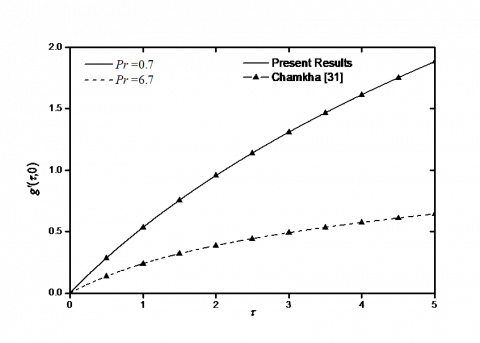Figure 2. Comparison between present results and Chamkha .

As we can see form this Fig., the results are found in a good agreement with these results.

4. Results and Discussion

In order to gain a clear physical insight of this investigation, the impact of the Darcy number Da, solid volume fraction parameter f, slip factors δ1 and δ2, and Biot number Bi on the profiles of nanofluid velocity components, pressure and temperature as well as the local skin-friction coefficients in the x and y orientations Cfx and Cfy, and the local Nusselt number Nux are presented in Figs. 3-8. The current numerical investigation is carried out for copper-water nanofluid as working fluid and the value of Prandtl number Pr of  base fluid (water) is kept constant at 6.2.

Figs. 3(a)-3(e) visualize the impact of the Darcy number on the profiles of nanofluid velocity components in x-orientation ${f}'(\tau ,\eta )$ and $g(\tau ,\eta )$, velocity component in y-orientation $h(\tau ,\eta )$, pressure $G(\tau ,\eta )$, and temperature $\theta (\tau ,\eta )$, respectively. It is noteworthy to mention from the definition of Da, that the value of Darcy number measures the extent of the permeability of porous medium, that is the existence of a porous medium in the flow provides resistance to flow, thus, this resistive force leads to a considerable decelerate the motion of the nanofluid along the stretched surface. This is appeared in Figs. 3(a)-3(e) by increasing the Darcy number Da from 0.001, 0.01,1 (very high permeability) to 10 (weak permeability) clearly induces a pronounced enhancement in the x-velocity components (${f}'$and g) and y-velocity component h, i.e., accelerates the flow. In addition, the variations in velocities are maximized some distance from the wall, towards the free stream. As such the nanofluid acceleration towards the edge of the boundary layer is less impeded by wall effects here. Also, evolution in Da implies to damp the Darcian drag force, due to the inverse relationship in Eqs. (10)-(13); porous drag force is therefore progressively lowered with an evolution in permeability, i.e., Darcy number, which serves to promote the flow velocities in the regime. Subsequently, these promotes in the flow have a tendency to a robust dwindling in both of temperature and pressure profiles. In addition, it is interesting to note that an increment in the values of Da are followed by corresponding rise in all hydrodynamics boundary layers and slight diminution in thermal boundary layers thickness.

## 31.png(a)

## 32.png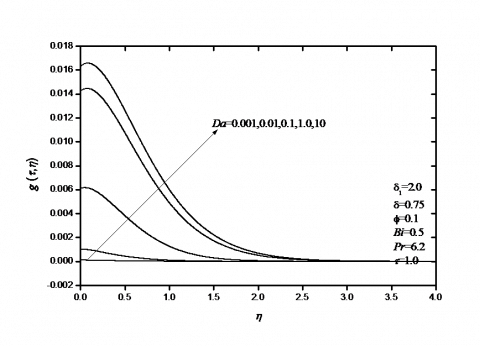(b)

## 33.png(c)

## 34.png(d)

## 35.png(e)

Figure 3. Impact of Darcy Number Da on (a) fluid velocity of x-orientation (${f}'$), (b) fluid velocity of x-orientation (g), (c) fluid velocity of y-orientation (h), (d) pressure profiles (G), (e) fluid temperature ($\theta$)

Figs 4(a)-4(d) demonstrate the impact of Darcy number Da upon variation in skin-friction coefficients in the x- and y- orientations Cfx and Cfy and local Nusselt number Nux, respectively, against the dimensionless time t. As seen from the definitions of Cfx, Cfy and Nux, they are directly proportional to ${f}''(\tau ,0)$,${g}'(\tau ,0)$,${h}'(\tau ,0)$and$-{\theta }'(\tau ,0)$, respectively. it is manifested from these Figs. that there are two opposite behaviors for the local skin-friction coefficients and local Nusselt number. These behaviors are clarified by the reduction in the skin-friction coefficient in the x-orientation ${f}''(\tau ,0)$ and a huge enhancement in either the skin-friction coefficients in the x- and y-orientations ${g}'(\tau ,0)$,${h}'(\tau ,0)$ and the Nusselt number $-{\theta }'(\tau ,0)$ as a result of rising the Darcy number Da.

## 41.png(a)

## 42.png(b)

## 43.png(c)

## 44.png(d)

Figure 4. Impact of Darcy Number Da on (a) the skin-friction coefficient in the x-orientation f''(τ,0), (b) skin-friction coefficient in the x-orientation g'(τ,0), (c) skin-friction coefficient in the y-orientation h'(τ,0), (d) wall heat transfer -θ'(τ,0)

This is due to the fact that the increment in the flow velocity components close to the wall with decreasing the nanofluid temperature as Da enlarges, causing the wall slope of the fluid linear velocity and the negative wall slope of the temperature profiles to grow. On other hand, both the local skin-friction coefficients and Nusselt number become lower at the dimensionless time t=0 and they are larger with high values of t.

Figs. 5(a)-5(e) depict the influences of slip factors δ1 and δ2 on dimensionless nanofluid velocity components, pressure and temperature profiles, respectively. It is apparent from these Figs. and based on the definition of slip ratio that an elevation in the slip factors δ1 and δ2 results in a considerable reduction in both the x-velocity component ${f}'$ and pressure profiles G, while a reverse trend occurred with the profiles of velocity of x-orientation (g), fluid velocity of y-orientation (h), and nanofluid temperature q. This is due to the fact that a rising the values of the velocity slip parameters have a tendency to accelerate the flow along the y-orientation which is inclined to the horizontal line, whereas a reverse trend occurred along the stretched surface in the x-orientation. This, in turn, produces a sufficient depress in x-velocity component and a strong promotes occurs in the fluid velocity profiles in y-orientation and temperature. There will be a corresponding increase in the hydrodynamics and thermal boundary layer thickness. These behaviors are clearly shown in Figures 5(a)-5(e).

## 51.png(a)

## 52.png(b)

## 53.png(c)

## 54.png(d)

## 55.png(e)

Figure 5. Impact of slip factor δ1 on (a) fluid velocity of x-orientation (${f}'$), (b) fluid velocity of x-orientation (g), (c) fluid velocity of y-orientation (h), (d) pressure profiles (G), (e) fluid temperature ($\theta$)

The variations of the skin-friction coefficients and local Nusselt number with different values of slip factors δ1 and δ2 are respectively, shown in Figs 6(a)-6(d). It is noteworthy to mention that an augmentation in the slip factors δ1 and δ2 implies a noticeable reduction in all the local skin-friction coefficient and Nusselt number. This result was predicted because the increment in the velocity slips elucidate a considerable acceleration in the flow along the y-orientation with maximization in the hydrodynamics and thermal boundary layers thickness, as depicted in Figures 5(a)-5(e).

## 61.png(a)

## 62.png(b)

## 63.png(c)

## 64.png(d)

Figure 6. Impact of slip factor δ1 on (a) the skin-friction coefficient in the x-orientation ${f}''(\tau ,0)$, (b) skin-friction coefficient in the x-orientation ${g}'(\tau ,0)$, (c) skin-friction coefficient in the y-orientation ${h}'(\tau ,0)$, (d) wall heat transfer $-{\theta }'(\tau ,0)$

Figs. 7(a)-7(e) elucidate the influences of solid volume fraction parameter f and Biot number Bi on the nanofluid velocity components, pressure and temperature profiles, respectively. it is noteworthy to mention that a raise in the solid volume fraction parameter f results in a considerable decrease in all the velocity components and pressure profiles. Moreover, the nanofluid temperature reduces near the stretched surface with the enhancement in the solid volume fraction parameter f, whereas the opposite behavior occurs with temperature after finite distance in the free stream. This consistency with the physical behavior that increasing the volume of nanoparticles implies a high thermal conductivity of nanofluid (see Table 1), consequently the thermal boundary layer thickness increases. The cause for this outcome is that an increment in the volume fraction produces a high-energy transport through the flow associated with the irregular motion of the ultrafine particles. On other side, the enhancement in the Biot number Bi causes a huge increase in either the fluid velocity of x-orientation (g), the fluid velocity of y-orientation (h) or temperature profiles. The cause for this behavior is that, a large value in Biot number yields a great surface convective which in turn provides more heat to the stretching surface and as a consequence of the temperature difference between the surface and the nanofluid will increase. As an outcome, the velocity as well as the wall temperature and thermal boundary layer thickness magnifies due to the enlargement in the values of Bi. Moreover, it is noteworthy to mention that the changes in Bi cause no effects on the behaviors in both the x-velocity components ${f}'$ and pressure profiles G due to the governing equations (11), (14) and (15) which are uncoupled from the other equations.

## 71.png(a)

## 72.png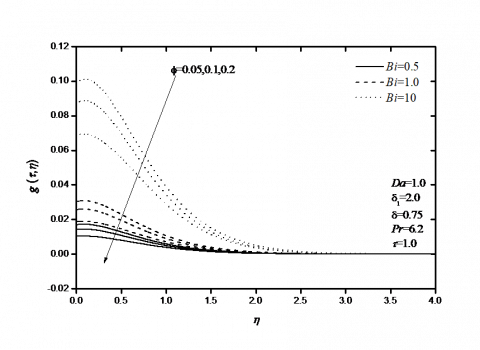(b)

## 73.png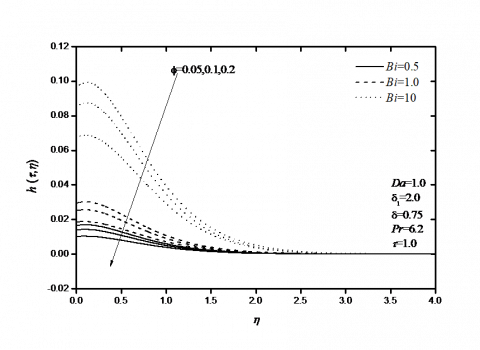(c)

## 74.png(d)

## 75.png(e)

Figure 7. Impact of solid volume fraction parameter f on (a) the fluid velocity of x-orientation (${f}'$), (b) fluid velocity of x-orientation (g), (c) fluid velocity of y-orientation (h), (d) pressure profiles (G), (e) fluid temperature ($\theta$)

Finally, Figs. 8(a)-8(d) exhibit the impact of the solid volume fraction f and Biot number Bi on the local skin-friction coefficients and Nusselt number. It is manifested that an increment in the solid volume fraction tends to damp the skin-friction coefficients and the Nusselt number, whilst the reverse behavior happens with the increase of Biot number Bi. This phenomenon is true even in the increase of solid volume fraction f, which, results in a increase in the thermal boundary layer thickness, associated with considerable decrease in the wall shear stress adjacent to the stretching surface with a decrease in the wall temperature gradient, and hence produces a sufficient reduction in the local Nusselt number. Furthermore, It is manifested that an increasing in the value of Biot number Bi has a tendency to increase both the local skin friction coefficients and local Nusselt number. The reason for this behavior is that as Bi increases, the cold nanofluid on stretched surface is convectively heated and thus, the flow velocity rises, which in turn enhances in the gradients of velocity components and temperature.

## 81.png(a)

## 82.png(b)

## 83.png(c)

## 84.png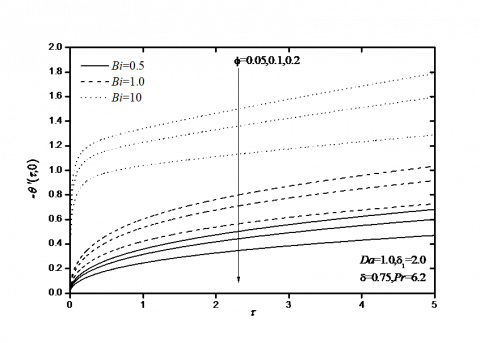(d)

Figure 8. Impacts of solid volume fraction parameter f and Biot number Bi on (a) the skin-friction coefficient in the x-orientation ${f}''(\tau ,0)$, (b) skin-friction coefficient in the x-orientation ${g}'(\tau ,0)$, (c) skin-friction coefficient in the y-orientation ${h}'(\tau ,0)$, (d) wall heat transfer $-{\theta }'(\tau ,0)$

5. Conclusions

This analysis is ensued to discuss the impacts of the anisotropic slip and convective boundary condition on the unsteady three-dimensional flow of nanofluid along an inclined stretching surface saturated with porous medium. The governing equations are solved by implicit numerical scheme of finite-difference type. The impacts of various significant parameters on flow and heat transfer are accomplished through graphs. From the computational data, it is concluded that there are two opposite behaviors for the local skin-friction coefficients and local Nusselt number as Darcy number increased. These behaviors are illustrated by the reduction in the skin-friction coefficient in the x-orientation and the enhancement in both the skin-friction coefficients in the y-orientations and local Nusselt number due to increases in Darcy number. In addition, it is found that all the local skin-friction coefficients and local Nusselt number were reduced as either of velocity slip factors or solid volume fraction parameter were increased. Furthermore, it is found that an increment in the Biot number implies a sufficient enhancement in both the local skin friction coefficients and local Nusselt number.

References

 Nield D.A., Bejan A. (2006). Convection in Porous Media. 3rd Ed., Springer, New York.

 Vafai K. (2005). Handbook of Porous Media. 2nd ed., Taylor & Francis, New York.

 Pop I., Ingham D.B. (2001). Convective Heat Transfer, Mathematical and Computational Modelling of Viscous Fluids and Porous Media. Pergamon, Oxford.

 Ingham D.B., Pop I. (2002). Transport Phenomena in Porous Media. eds. Pergamon, Oxford.

 Das S.K., Choi S.U.S., Yu W., Pradeep T. (2007). Nanofluids-Science and Technology. John Wiley & Sons Publishers, Hoboken.

 Choi S.U.S. (1995). Enhancing thermal conductivity of fluids with nanoparticle. in, D.A. Siginer, H.P. Wang (Eds.), developments and applications of non-newtonian flows, ASME FED,Vol. 231, pp. 99-105.

 Buongiorno J. (2006). Convective transport in nanofluids, ASME J. Heat Transf., Vol. 128, pp. 240-250. DOI: 10.1115/1.2150834

 Duangthongsuk W.S., Wongwises. (2008). Effect of thermophysical properties models on the predicting of the convective heat transfer coefficient for low concentration nanofluid, International Communications in Heat and Mass Transfer, Vol. 35, pp. 1320-1326. DOI: 10.1016/j.icheatmasstransfer.2008.07.015.

 Nield D.A., Kuznetsov A.V. (2009). Thermal instability in a porous medium layer  saturated by a nanofluid, Int. J. Heat and Mass Transfer, Vol. 52, pp. 5796-5801. DOI: 10.1016/j.ijheatmasstransfer.2009.07.023

 Das S.K., Putra N., Thiesen P., Roetzel W. (2003). Temperature dependence of thermal conductivity enhancement for nanofluids, J. Heat Transfer, Vol. 125, pp. 567-574. DOI: 10.1115/1.1571080

 Tiwari R.K., Das M.K. (2007). Heat transfer augmentation in a two-sided lid-driven differentially heated square cavity utilizing nanofluids, Int. J Heat Mass Tranfer, Vol. 50, pp. 2002-2018. DOI: 10.1016/j.ijheatmasstransfer.2006.09.034

 Rashad A.M., EL-Hakiem M.A., Abdou M.M.M. (2011). Natural convection boundary layer of a non-Newtonian fluid about a permeable vertical cone embedded in a porous medium saturated with a nanofluid, Computers and Mathematics with Applications, Vol. 62, pp. 3140-3151. DOI: 10.1016/j.camwa.2011.08.027

 Chamkha A.J., Modather M., EL-Kabeir S.M.M., Rashad A.M. (2012). Radiative effects on boundary-layer flow of a nanofluid on a continuously moving or fixed permeable surface, Recent Patents on Mechanical Engineering, Vol. 5, No. 3, pp. 176-183. DOI: 10.2174/2212797611205030176

 Hady F.M., Ibrahim F.S., Abdel-Gaied S.M. (2012). Radiation effect on viscous flow of a nanofluid and heat transfer over a nonlinearly stretching sheet, Nanoscale Res. Lett., Vol. 7, pp. 229-236. DOI: 10.1186/1556-276X-7-229

 Chamkha A.J., Rashad A.M. (2012). Natural convection from a vertical permeable cone in nanofluid saturated porous media for uniform heat and nanoparticles volume fraction fluxes, Int. J Numerical Methods for Heat and Fluid Flow, Vol. 22, No. 8, pp. 1073-1085. DOI: 10.1108/09615531211271871

 Chamkha A.J., Abbasbandy S., Rashad A.M., Vajravelu K. (2012). Radiation effects on mixed convection over a wedge embedded in a porous medium filled with a nanofluid, Transport in Porous Medium, Vol. 91, pp. 261-279. DOI: 10.1007/s11242-011-9843-5

 Chamkha A.J., Abbasbandy S., Rashad A.M., Vajravelu K. (2013). Radiation effects on mixed convection about a cone embedded in a porous medium filled with a nanofluid, Meccanica, Vol. 48, No. 2, pp. 275-285. DOI: 10.1007/s11012-012-9599-1

 Bég O.A., Ferdows M. (2013). Explicit numerical simulation of magnetohydrodynamic nanofluid flow from an exponential stretching sheet in porous media, Appl. Nanosci., pp. 1-15. DOI: 10.1007/s13204-013-0275-0

 Chamkha A.J, Rashad A.M., Aly A.M. (2013). Transient natural convection flow of a nanofluid over a vertical cylinder, Meccanica, Vol. 48, No. 1, pp. 71-81. DOI: 10.1007/s11012-012-9584-8

 Ferdows M., Khan M.S., Bég O.A., Azad M.A.K., Alam M.M. (2013). Numerical study of transient magnetohydrodynamic radiative free convection nanofluid flow from a stretching permeable surface, Proc IMechE, Part E, J Process Mechanical Engineering. Epub ahead of print 25 September 2013, DOI: 10.1177/0954408913493406

 Noghrehabadi A., Behseresht A., Ghalambaz M., Behseresht J. (2013). Natural convection flow of nanofluids over a vertical cone embedded in a non-Darcy porous medium, J. Thermophys. Heat Transf., Vol. 27, pp. 334-341. DOI: 10.2514/1.T3965

 Behseresht A., Noghrehabadi A., Ghalambaz M. (2014). Natural convection heat and mass transfer from a vertical cone in porous media filled with nano fluids using the practical ranges of nano fluids thermophysical properties, Chem. Eng. Res. Des., Vol. 92, pp. 447-452. DOI: 10.1016/j.cherd.2013.08.028

 Rashad A.M., Abbasbandy S., Chamkha A.J. (2014). Non-Darcy natural convection from a vertical cylinder embedded in a thermally stratified and nanofluid-saturated porous media, ASME, Journal of Heat Transfer, Vol. 136, No. 2, DOI: 10.1115/1.4025559

 Rajesh V., Bég O.A., Mallesh M.P. (2014). Transient nanofluid flow and heat transfer from a moving vertical cylinder in the presence of thermal radiation, Numerical study. Proceedings of the Institution of Mechanical Engineers. Part N, Journal of Nanoengineering and Nanosystems. DOI: 10.1177/1740349914548712

 EL-Kabeir S.M.M., Chamkha A.J., Rashad A.M. (2014). The effect of thermal radiation on non-Darcy free convection from a vertical cylinder embedded in a nanofluid porous media, Journal of Porous Media, Vol. 17, No. 3, pp. 269-278. DOI: 10.1615/JPorMedia.v17.i3.70

 EL-Kabeir S.M.M., Modather M., Rashad A.M. (2015). Effect of thermal radiation on mixed convection flow of a nanofluid about a solid sphere in a saturated porous medium under convective boundary condition, Journal of Porous Media, Vol. 18, No. 6, pp. 569-584. DOI: 10.1615/JPorMedia.v18.i6.20

 Nasrin R., Alim M.A. (2015). Thermal performance of nanofluid filled solar flat plate collector, International Journal of Heat and Technology, Vol. 33, No. 2, pp. 17-24. DOI: 10.18280/ijht.330203

 Rajput G.R., Krishnaprasad J.S.V.R., Timol M.G. (2016). Group theoretic technique for MHD forced convection laminar boundary layer flow of nanofluid over a moving surface, International Journal of Heat and Technology, Vol. 34, No. 1, pp. 1-6. DOI: 10.18280/ijht.340101.

 Pragya V.R. (2016). Boundary layer flow of silver and titaniumoxide nanofluids over vertical stretching sheet, International Journal of Heat and Technology, Vol. 34, No. 3, pp. 371-376. DOI: 10.18280/ijht.340304

 Blottner F.G. (1970). Finite-difference methods of solution of the boundary-layer equations, AIAA Journal, Vol. 8, pp. 193-205. DOI: 10.2514/3.5642

 Chamkha A.J. (2000). Transient hydromagnetic three-dimensional natural convection from an inclined stretching permeable surface, Chemical Engineering Journal, Vol. 76, pp. 159-168. DOI: 10.1016/S1385-8947(99)00120-5